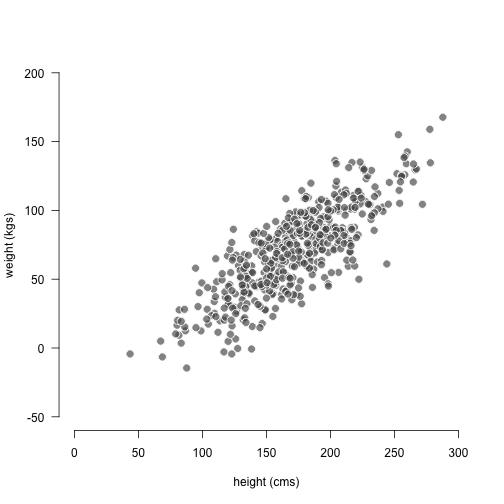# Football-shaped scatterplot

##### Posted on March 23, 2016

Using R to plot a football-shaped scatter diagram.## Introduction

In all my Introduction to Statistics courses I need to discuss topics such as association between two quantitative variables, correlation coefficient, and of course scatter diagrams.

A particular type of scatter diagrams involves what some of my colleagues at UC Berkeley call a football-shaped scatterplot. This is the generic term we used to indicate that two (quantitative) variables X and Y, supposedly distributed in fairly normal way, have a linear association. Moreover, the diagram shows homoscedasticity (same amount of vertical spread).

How can you graph such a scatterplot in R? One approach that you can use is to generate variables X and Y that follow a bivarate normal distribution. This can be done with the function mvrnorm(), from the R package "MASS".

The following code allows you to generate a bivariate normal distribution based on summary statistics for variables X and Y. You need to specify the univariate means, standard deviations (and variances), as well as the desired correlation coefficient. Taking into account the provided inputs, a vectors of means, and a variance-covariance matrix are obtained with mvrnorm(). And finally a scatterplot is produced with plot():Published in categories how-to  Tagged with scatter  plot